Need help with Manim-Tutorial?
Click the “chat” button below for chat support from the developer who created it, or find similar developers for support.malhotra5475 Stars78 Forks151 Commits9 Opened issuesDescription

A tutorial for manim, a mathematical animation engine made by 3b1b!
?

Need anything else?Contributors list# 176,225
Python
graphic...
126 commits# 312,157
graphic...
c-sharp
Dart
guideli...
2 commits# 533,677
Python
graphic...
2 commits# 594,311
Python
graphic...
1 commit# 155,745
Python
TeX
gist
graphic...
1 commit# 234,854
graphic...
Svelte
PureScr...
sed
1 commit

Manim-Tutorial

A tutorial for manim, a mathematical animation engine made by 3b1b for Python.

Requirements

• Python 3.7 (I managed to run it on version 3.6.7, so I'm guessing 3.6 and above works)
• An operating system (Linux, Windows or Macintosh)

Installations

Lets first get the manim repo and python dependencies using the terminal -

OPTIONS: Use either

Command 2
or
Command 3
below. For
Command 3
, you need to be inside the manim directory.
git clone https://github.com/3b1b/manim.git  #Command 1
pip3 install manimlib                        #Command 2
python3 -m pip install -r requirements.txt   #Command 3

NOTE: Although it is highly recommended, the following system installations are only required if you want to use

LaTeX

Linux Users

These are the final system requirements:

Cairo
,
Latex
,
ffmpeg
and
sox
.
sudo apt install ffmpeg sox
sudo apt-get install libcairo2-dev libjpeg-dev libgif-dev
sudo apt install texlive-latex-base texlive-full texlive-fonts-extra

Windows Users

!!!to my knowledge manim only works with python 3.7 (yet)!!!

• install Sox

• install ffmpeg

• install miktex

• install pycairo, this can be pycairo‑1.19.1‑cp37‑cp37m‑win_amd64.whl or pycairo‑1.19.1‑cp37‑cp37m‑win32.whl depending on your achitecture and python version. (The 37 stands for python 3.7)

• install pycairo using

pip install [your-pycairo-whl]
in the directory where the pycairo wheel is located.
• download manim zip file from the github repo and extract it

make a new venv, if you want to

delete pycairo from the requirentments.txt

run

pip install -r requirentments.txt

Common Problems

• Problem #1: Cairo System requirement People are sometimes unable to install

Cairo
through the terminal. But, it is possible to install it using the Python.
bash
pip3 install pycairo

• Problem #2: Exception: Latex error converting to dvi. See log output above or the log file This error can be frustrating. Especially when you don't know what to install. But if you followed my installation guide, this error is not due to missing a system requirement. Rather, there is a problem with the code.

• Problem #3: No module named manim This error occurs when you use the command to run a manim project when your not in the parent directory. Make sure that your current directory is in manim, and no other sub directory.

Using Virtual Box

If you still face problems in installation, you can use manim on Virtual Box. It creates a virtual Ubuntu os on your host os. All the dependencies are pre-installed so that you are directly use manim.

1. Install VirtualBox.
3. Install the file
4. Use
fossee
5. Clone manim repository

git clone https://github.com/3b1b/manim.git
cd manim
python3 manim.py example_scenes.py SquareToCircle -pl

Running Manim Projects

Easy way to test whether all your installations are working is by running the command below

bash
python3 -m manim example_scenes.py SquareToCircle -pl

If it worked, then congratulations! Now you can run manim programs and get started with making animations. Now, this will be the general command to run all manim projects

python
python3 -m manim pythonFile.py className -args

NOTE 1: Your videos that you make are saved in the folder called videos. \ NOTE 2: The command for running the manim programs should only be run in the parent directory.

classNames

Manim programs have a certain structure. The Python program file requires you to make classes for all your series of animations. If you make more than a few classes, you have to run commands for every class you make. Seperate videos are made for every class.

Args

Args are a list of arguements that can be stated when running the program. The most important agruements and it's explanations are provided in the GO TO GUIDE. I recommend to look at it later, and start with the tutorial.

Tutorial!

Finally we can start. In this tutorial, we will learn by doing.

Basics

from manimlib.imports import *

class Shapes(Scene): def construct(self): ######Code###### #Making shapes circle = Circle() square = Square() triangle=Polygon(np.array([0,0,0]),np.array([1,1,0]),np.array([1,-1,0]))

#Showing shapes
self.play(ShowCreation(circle))
self.play(GrowFromCenter(square))
self.play(Transform(square,triangle))

We will break this into parts: * Import: The import in this code is the import we will use in all manim projects. It has almost all the imports we will ever require * Class: For running animations, you have to make a class that has a base class from manim. * Method: The construct method is special to manim. Manim calls on construct for creating animations. Therefore, every class that runs manim has to have this method. * Code: You don't have to fully understand how the code works yet. But you can see that you first define your animations, and then you display it. You can experiment with the order in which you define and display.

NOTE: If you recall, to run this animation, you would run the following in the terminal -

bash
python3 -m manim fileName.py Shapes -pl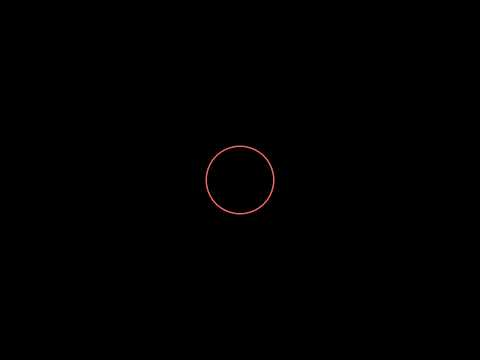Shapes

from manimlib.imports import *
from math import cos, sin, pi

class Shapes(Scene): def construct(self): #######Code####### #Making Shapes circle = Circle(color=YELLOW) square = Square(color=DARK_BLUE) square.surround(circle)

rectangle = Rectangle(height=2, width=3, color=RED)
ellipse=Ellipse(width=5, height=3, color=DARK_BLUE)

pointers = []
for i in range(8):
pointers.append(Line(ORIGIN, np.array([cos(pi/180*360/8*i),sin(pi/180*360/8*i), 0]),color=YELLOW))

#Showing animation
self.play(Transform(square, rectangle))
self.play(Transform(ring,ring2))
self.play(Transform(ring2, ring))
self.wait(2)

After looking at a lot of pieces of code in this tutorial, you will eventually familiarize yourself with manim. So lets start!

Our focus is going to shift from understanding the structure of our code, to understanding the code itself. The first import statement imports many of the classes we will use.

The section for making shapes creates shapes that can be used in manim. You can define it's size, color,etc. You will see some methods such as surround or FadeOut, we wil classify them later. The code is simple enough to read, most of it looks like English.

The section for showing the animaton displays the shapes, as specified in the code. Let's look at the what the code offers.

Shapes: The shapes defined in manim are known as mobjects. Manim has this classification for objects other than shapes. Keep reading for the formal definition of a mobject. * Square * Circle * Rectangle * Lines * Annulus

Animations: These are animations that apply to objects known as mobjects. Mobjects are objects defined by manim. Manim creates these objects specifically, so that you can apply any animations or other special manim methods to them. * FadeIn * Transform * FadeOut * GrowFromCenter

Adding: These are some of the methods for adding mobjects or playing Animations on mobjects. Note: If you play an animation, you don't have to add it to the screen. The animation does it for you. * Play * Add

In this code, I specifically included an example that I found useful to know.

python
pointers.append(Line(ORIGIN, np.array([cos(pi/180*360/8*i),sin(pi/180*360/8*i), 0]),color=YELLOW))
I am appending mobjects into an list. This way I can manipulate the mobjects in the list. However, some manim methods such as FadeOut() can't take multiple mobjects at once. This makes it hard to do multiple tasks with less lines of code. We will take a look at a way to overcome that problem later. Although, some methods do however take multiple mobjects.

For example: self.add() took the list. However, you have to unpack the list first.

python
Here, mobjects in the list pointers, we unpacked and passed as arguments to add(). Notice the syntax for doing so. We put * before the list.

Last note. If you realized, the base class of the class above was Scene. This is provided by manim. Using it, we can access methods pertaining to manim. Manim also has many other base classes that we can use. If you realize, the lines of code below come from the base class.

python
self.play()
There are other bases classes we will explore for making Graphs, 3D Scenes,etc.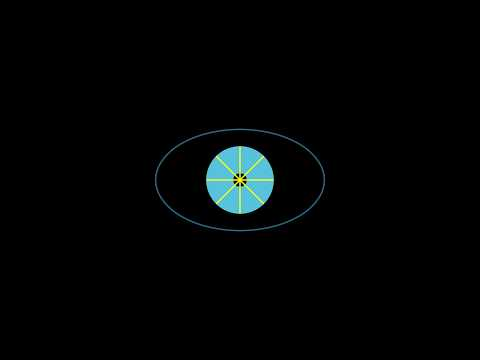Text

from manimlib.imports import *

class makeText(Scene): def construct(self): #######Code####### #Making text first_line = TextMobject("Manim is fun") second_line = TextMobject("and useful") final_line = TextMobject("Hope you like it too!", color=BLUE) color_final_line = TextMobject("Hope you like it too!")

#Coloring

#Position text
second_line.next_to(first_line, DOWN)

#Showing text
self.wait(1)
self.play(Write(first_line), Write(second_line))
self.wait(1)
self.wait(1)
self.play(Transform(final_line, color_final_line))
self.wait(2)

Hopefully, most of the code makes sense. In this section I'll introduce a new mobject known as TextMobject. It is used to store text. It is particulary useful because it helps you position text on the screen and you can use the animation write().

I also included a nice coloring tool, setcolorby_gradient. You can pass constants in Manim such as BLUE or PURPLE. To pass a custom color you can specify the hex code of the color instead of using Manim color constants.

TextMobjects will be used later on to write good looking math equations.Math Equations

from manimlib.imports import *

class Equations(Scene): def construct(self): #Making equations first_eq = TextMobject("$$J(\theta) = -\frac{1}{m} [\sum_{i=1}^{m} y^{(i)} \log{h_{\theta}(x^{(i)})} + (1-y^{(i)}) \log{(1-h_{\theta}(x^{(i)}))}]$$") second_eq = ["$J(\theta_{0}, \theta_{1})$", "=", "$\frac{1}{2m}$", "$\sum\limits_{i=1}^m$", "(", "$h_{\theta}(x^{(i)})$", "-", "$y^{(i)}$", "$)^2$"]

second_mob = TextMobject(*second_eq)

for i,item in enumerate(second_mob):
if(i != 0):
item.next_to(second_mob[i-1],RIGHT)

eq2 = VGroup(*second_mob)

des1 = TextMobject("With manim, you can write complex equations like this...")
des2 = TextMobject("Or this...")
des3 = TextMobject("And it looks nice!!")

#Coloring equations

#Positioning equations
des1.shift(2*UP)
des2.shift(2*UP)

#Animating equations
self.play(Write(des1))
self.play(Write(first_eq))
self.play(ReplacementTransform(des1, des2), Transform(first_eq, eq2))
self.wait(1)

for i, item in enumerate(eq2):
if (i&lt;2):
eq2[i].set_color(color=PURPLE)
else:
eq2[i].set_color(color="#00FFFF")

self.wait(1)
self.wait(2)

Here, we will look at very important concepts that will help when using Manim.

That looks long, but it's very simple. Here I have provided 2 ways of making equation and displaying it to the screen. If you remember, we installed some latex system requirements. We will use LaTex to make our equations look nice.

LaTex will take it's own tutorial. However, you don't need to know a lot of LaTex. I will introduce some rules that will help you write any math equation. Notice that equations are specified in TextMobjects.

LaTex: When making an equation, the general rule is to put a $at the start and end of the text. For example: python text = TextMobject("This is text") #Normal text equation = TextMobject("$X$") #This is an equation X Now for the fun part. In LaTex, you can represent symbols using a backslash and a keyword. THis include theta, alpha, summation, etc. In Manim, it is similar. theta = TextMobject("$\\theta$") Notice, in Manim, you specify symbols by putting 2 backslash before the keyword. Finally, the I will introduce the syntax for adding subscripts and superscripts. Here is the syntax for superscripts. superScript_equation = TextMobject("$r^{\\theta}$") The ^ symbol signifies superscript. We put the symbol theta as the superscript. Also, when specifying superscript the {} brackets are not displayed in the equation. They help group all the elements you want to add to the superscript. For subscripts, it is similar. subScript_equation = TextMobject("$\\theta_{1}$") This is theta subscript 1. The _ signifies subscript. Like usual, the {} brackets aren't displayed in the equation. For more symbol options, go to the resources section. Now, we will look at a complex way of writing equations using VGroup. Let's look at what a VGroup is. A VGroup is a list of mobjects who to which you can apply animations all at once. For example, if you have a list of circles, you could transform all of them into squares, by only transforming the VGroup. Capabilities: You can animate all the mobjects at once, you can animate specific mobjects by indexing them in their list. Let's look at the example where we make a VGroup for the math equation. second_eq = ["$J(\\theta_{0}, \\theta_{1})$", "=", "$\\frac{1}{2m}$", "$\\sum\\limits_{i=1}^m$", "(", "$h_{\\theta}(x^{(i)})$", "-", "$y^{(i)}$", "$)^2$"] Click for results on YouTube: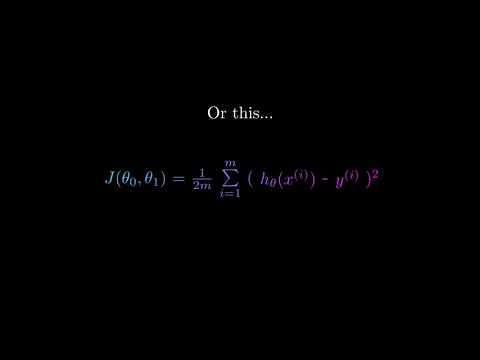Graphing from manimlib.imports import * import math class Graphing(GraphScene): CONFIG = { "x_min": -5, "x_max": 5, "y_min": -4, "y_max": 4, "graph_origin": ORIGIN, "function_color": WHITE, "axes_color": BLUE } def construct(self): #Make graph self.setup_axes(animate=True) func_graph=self.get_graph(self.func_to_graph,self.function_color) graph_lab = self.get_graph_label(func_graph, label = "x^{2}") func_graph_2=self.get_graph(self.func_to_graph_2,self.function_color) graph_lab_2 = self.get_graph_label(func_graph_2, label = "x^{3}") vert_line = self.get_vertical_line_to_graph(1,func_graph,color=YELLOW) x = self.coords_to_point(1, self.func_to_graph(1)) y = self.coords_to_point(0, self.func_to_graph(1)) horz_line = Line(x,y, color=YELLOW) point = Dot(self.coords_to_point(1,self.func_to_graph(1))) #Display graph self.play(ShowCreation(func_graph), Write(graph_lab)) self.wait(1) self.play(ShowCreation(vert_line)) self.play(ShowCreation(horz_line)) self.add(point) self.wait(1) self.play(Transform(func_graph, func_graph_2), Transform(graph_lab, graph_lab_2)) self.wait(2) def func_to_graph(self, x): return (x**2) def func_to_graph_2(self, x): return(x**3) By now you should be able to identify similar patterns when coding with Manim. The config dictionary, specifies various parameters for graphing: the axis size, axis color or graph colors. The exact parameters are pretty self explanatory and are specified below. To make a graph, you have to specify a method that returns the y value for evey x value inupt. This is specified in the method functograph. The method get_graph creates a mobject out of the previous method, which can be manipulated. Note, that the graph method only specifies what the graph should look like given a point. But, the extent of how much is displayed (like from -5 to 5 on the x axis) is determined by the CONFIG dictionary. Here is the default dictionary Manim uses for graphing. python CONFIG = { "xmin": -1, "xmax": 10, "xaxiswidth": 9, "xtickfrequency": 1, "xleftmosttick": None, # Change if different from xmin "xlabelednums": None, "xaxislabel": "$x$", "ymin": -1, "ymax": 10, "yaxisheight": 6, "ytickfrequency": 1, "ybottomtick": None, # Change if different from ymin "ylabelednums": None, "yaxislabel": "$y\$", "axescolor": GREY, "graphorigin": 2.5 * DOWN + 4 * LEFT, "excludezerolabel": True, "numgraphanchorpoints": 25, "defaultgraphcolors": [BLUE, GREEN, YELLOW], "defaultderivativecolor": GREEN, "defaultinputcolor": YELLOW, "defaultriemannstartcolor": BLUE, "defaultriemannendcolor": GREEN, "areaopacity": 0.8, "num_rects": 50 }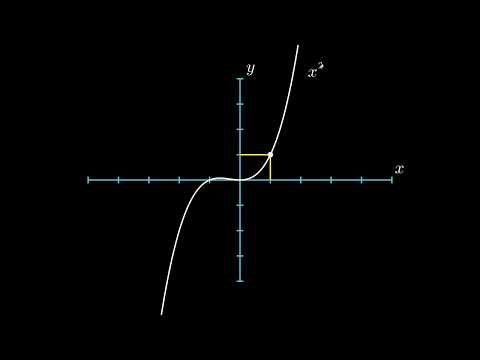3D Graphing

Spheres and more

python from manimlib.imports import *

class ThreeDObjects(SpecialThreeDScene): def construct(self): sphere = self.get_sphere() cube = Cube() prism = Prism() self.play(ShowCreation(sphere)) self.play(ReplacementTransform(sphere, cube)) self.play(ReplacementTransform(cube, prism)) self.wait(2)

Custom Graphs

from manimlib.imports import *

class ThreeDSurface(ParametricSurface):

def __init__(self, **kwargs):
kwargs = {
"u_min": -2,
"u_max": 2,
"v_min": -2,
"v_max": 2,
"checkerboard_colors": [BLUE_D]
}
ParametricSurface.__init__(self, self.func, **kwargs)

def func(self, x, y):
return np.array([x,y,x**2 - y**2])

class Test(ThreeDScene):

def construct(self):
self.set_camera_orientation(0.6, -0.7853981, 86.6)

surface = ThreeDSurface()
self.play(ShowCreation(surface))

d = Dot(np.array([0,0,0]), color = YELLOW)
self.play(ShowCreation(d))

self.wait()
self.move_camera(0.8*np.pi/2, -0.45*np.pi)
self.begin_ambient_camera_rotation()
self.wait(9)

Alright! Finally some 3D graphs. So, the first ThreeDSurface inherits from parametric surfaces. This will be used to define our 3D graph in terms of a mathematical equation. The kwargs parameter are just some tweaks that change the color of the the graph, or how much of the graph should be rendered. The method func defines the function. It returns the z given the x and y parameters (which are required for 3D graphs).

The ThreeDSurface is called in the Test class and is manipulated like a mobject. You render the 3D graph like any other mobject in manim.

A continuation of this tutorial will follow to explain how the camera works. For now, the camera is basically your eyes.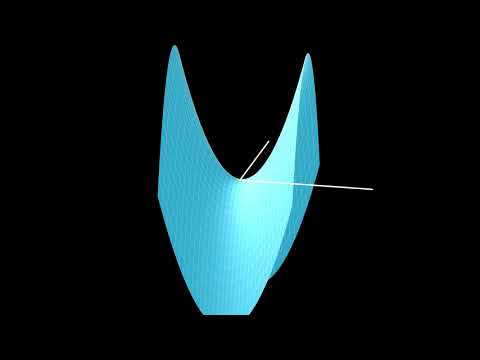Images

Manim has a mobject made for images. You can resise them, invert their colors, etc by using Manim methods.

from manimlib.imports import *

class Images(Scene): def construct(self): img = ImageMobject('pathToIm.png') img.scale(2) # Resize to be twice as big img.shift(2 * UP) # Move the image

self.play(ShowCreation(img))  # Display the image

Alternatively, you could load the image using OpenCV or PIL, and then display the image using Manim.

from manimlib.imports import *
import cv2

class Images(Scene): def construct(self): img = cv2.imread('pathToImg.png') imMob = ImageMobject(img) # Makes an image mobject of existing image

imMob.scale(2)
imMob.shift(2 * UP)

self.play(ShowCreation(imMob))

Putting it together

Manim is extremely powerful, and is capable of creating high quality graphics. You can make your animations using graphics and then overlay your voice over the video.

If you were able to follow this tutorial successfully, then Congrats! Hopefully you can proficiently use Manim!

Further Work

I am missing a lot of aspects behind this powerful library after reverse engineering manim. There are things such as 3D scenes that still need to be documented. But hopefully this guide will cater to your basic needs.

Acknowledgments

• 3 Blue 1 Brown: The creator of this engine who uses it for creating cool math videos. Visit his YouTube channel and manim repo.
• Todd Zimmerman: Recently made a new documentation of manim in Python3.7.